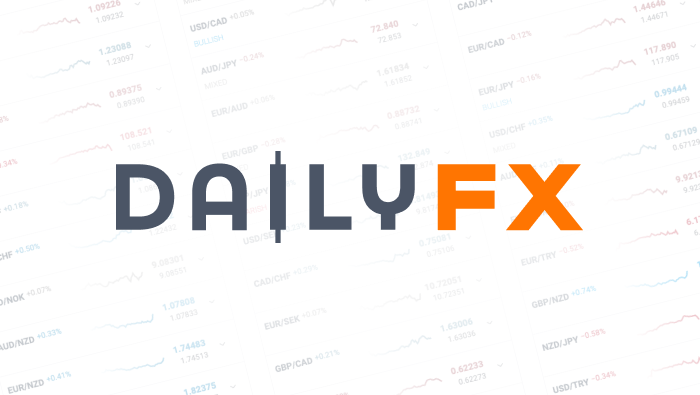We use a range of cookies to give you the best possible browsing experience. By continuing to use this website, you agree to our use of cookies.

EUR/USD
Mixed
Low
High
Long Short

Note: Low and High figures are for the trading day.

Data provided by
Oil - US Crude
Bearish
Low
High
Long Short

Note: Low and High figures are for the trading day.

Data provided by
Wall Street
Mixed
Low
High
Long Short

Note: Low and High figures are for the trading day.

Data provided by
Gold
Bullish
Low
High
Long Short

Note: Low and High figures are for the trading day.

Data provided by
GBP/USD
Bearish
Low
High
Long Short

Note: Low and High figures are for the trading day.

Data provided by
USD/JPY
Mixed
Low
High
Long Short

Note: Low and High figures are for the trading day.

Data provided by# How the Key Fibonacci Level Can Lock-In Great Risk: Reward TradesTyler Yell, CMT, Currency Strategist

Talking Points:

• Fibonacci Basics
• The Key Fibonacci Level
• Great Risk: Reward Baked In To The Key Level

This article builds upon the prior Fibonacci Article designed to show you how to find high-impact price zones on the chart. The article used real-time examples where multiple Fibonacci levels in a common area resulted in a price explosion off that zone, along with a handful of charts. Today’s article will help you use the critical Fibonacci Level to derive a strong risk: reward ratio.

Fibonacci Basics

You may be familiar with the Fibonacci numbers, but most traders are concerned or

interested in the Fibonacci Ratios derived off the Fibonacci numbers. The number set is derived by starting with 0 and then adding the next whole integer, which is 1 to the prior number0, to give us the second number, 1. Proceeding with that logic, you could take 1 and add it again to the prior number, which is 1 and get 2. Add 2 to the prior integer of 1, you get three and so on and so forth.

Fibonacci sequence courtesy of The Rabbit Hole of Fibonacci by James StanleyThe ratios come together by looking at the ratios of the numbers within the Fibonacci sequence. Taking the Fibonacci sequence number of 13, you can divide it by the following 3 numbers to get critical ratio points. 13÷21=.61904, 13÷34=0.3823…, & 13÷55=0.2363... The first example will bring you the Golden Ratio any time you divide one Fibonacci number by the next Fibonacci number in the sequence.

If you’d like a video course on Fibonacci to explain the concept another way, register here for free.

The Key Fibonacci Level

You just learned that Fibonacci numbers are achieved by adding the number 1 to the prior number, zero, and then repeating into infinity to obtain the next Fibonacci number. While there are a handful of Fibonacci ratios, the most critical one is the .618 ratio and its inverse of 1.618 for price projections. As traders, the more useful information is to gather the ratios from the Fibonacci sequence as explained in the article, Using Fibonacci as a Leading Indicator, to point out critical levels to confirm or invalidate your trade.

Learn Forex: USDOLLAR INDEX Has Turned Sharply Off the Golden RatioPresented by FXCM’s Marketscope Charts

Looking above, you can see that the long-term chart of the USDOLLAR index has had two key pivots. Both pivots were corrective pivots off of the Golden Ratio of 61.8%. The most recent Fibo Pivot for USDOLLAR index pivot was in late January 2014, which marked the high for 2014 so far. Naturally, you can see retracements off the other Fibonacci levels, like the 23.6 or 38.2%, but the strongest moves tend to happen off deep retracements to the 61.8%.

Great Risk: Reward Baked In To the Key Level

Have you read our FREE Traits of Successful Traders Report? If not, there is one thing you should learn before you put on yourr next trade that we found unsuccessful traders were doing over and over again. Few found that many traders were stacking the odds against them because they were losing more money on losing trades than they made on winning trades. Therefore, we reasoned, traders should look to risk at most, half of their anticipated reward and ideally less.

Watch the FXCM EXPO Video from Our Quantitative Strategist on This Breakthrough FindingThe video goes on to explain that risking more than you seek on any trade puts the odds of trading against you. However, if you can look to gain, at least as much as you’re looking to risk, trading becomes a numbers gain. From this vantage point, you simply look for trades with a good edge, which can be found with a 61.8% retracement.A 1-to-1 or greater risk: reward ratio has dramatic effects on a trader’s expectancy. As you can imagine, a 61.8% retracement cuts a range into two-thirds, which is known as the Golden Section. Therefore, you can look to enter on a 61.8% retracement with a stop below the prior low and a target at or above the high for a 1:2 risk: reward ratio or better. The example below shows entering into the direction of the trend (short), after a deep retracement with a stop above the prior pivot high and a target at the prior low.

Learn Forex: GBPJPY Risk: Reward Ratio with Golden RatioPresented by FXCM’s Marketscope Charts